WHCSRL 技术网

# 【初识c语言】（2）

## 1.1 常量

### 2）实型常量

1）十进制小数形式，由数字和小数点组成。如123. 456,0. 345,- 56.79,0.0,12.0等。
2）指数形式，如12. 34e3(代表12. 34X103)，0.145E- 25(代表0. 145X10-25)等。由于在计算机输人或输出时无法表示上角或下角，故规定以字母e或E代表以10为底的指数。但应注意: e或E之前必须有数字，且e或E后面必须为整数。如不能写成e4,12e2. 5。

### 3）字符常量

#### 1）普通字符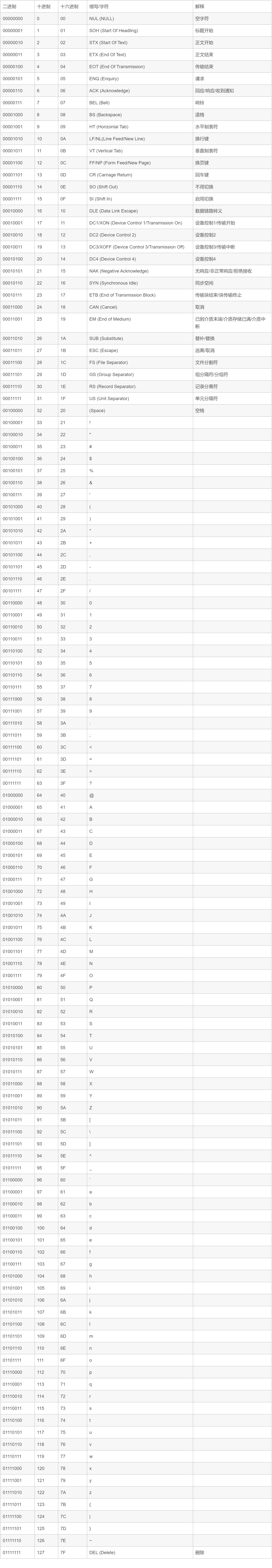#### 2）转义字符

"一个双撇号(")输出双撇号字符”
?一个问号(?)输出问号字符?

退格( backspace)将光标当前位置后退一个字符
f换页(form feed)将光标当前位置移到下一页的开头
n换行将光标当前位置移到下一-行的开头

v垂直制表符将光标当前位置移到下一个垂直制表对齐点
o,oo或ooo其中o代表一个八进制数字与该八进制码对应ASCII字符与该八进制码对应的字符
xh[h…]其中h代表一个十六进制数字与该十六进制码对应的ASCII 字符与该十六进制码对应的字符

### 5）符号常量

`# define PI 3. 1416`

## 1.2 变量

### 1）变量的声明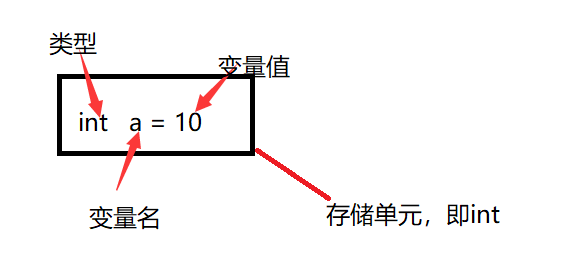### 2）赋值和初始化

#### 1)赋值

`int s=10;`

#### 2）初始化

`int a,b;`

## 2.1 运算符

C语言提供了以下运算符:

### 2）算术运算符

+把两个操作数相加A + B 将得到 30
-从第一个操作数中减去第二个操作数A - B 将得到 -10
*把两个操作数相乘A * B 将得到 200
/分子除以分母B / A 将得到 2
%%%%取模运算符，整除后的余数B %%%% A 将得到 0
++自增运算符，整数值增加 1A++ 将得到 11

（++），（- -）运算符

`#include<stdio.h>`
`int main()`
`{`
`int c;`
`int a = 10;`
`c = a++;`
```printf("先赋值后运算： ");```
```printf("Line 1 - c 的值是 %%%%d ", c );```
```printf("Line 2 - a 的值是 %%%%d ", a );```
`a = 10;`
`c = a--;`
```printf("Line 3 - c 的值是 %%%%d ", c );```
```printf("Line 4 - a 的值是 %%%%d ", a );```
```printf("先运算后赋值： ");```
`a = 10;`
`c = ++a;`
```printf("Line 5 - c 的值是 %%%%d ", c );```
```printf("Line 6 - a 的值是 %%%%d ", a );```
`a = 10;`
`c = --a;`
```printf("Line 7 - c 的值是 %%%%d ", c );```
```printf("Line 8 - a 的值是 %%%%d ", a );```
`}`

Line 1 - c 的值是 10
Line 2 - a 的值是 11
Line 3 - c 的值是 10
Line 4 - a 的值是 9

Line 5 - c 的值是 11
Line 6 - a 的值是 11
Line 7 - c 的值是 9
Line 8 - a 的值是 9

### 3）关系运算符

==检查两个操作数的值是否相等，如果相等则条件为真。(A == B) 不为真。
!=检查两个操作数的值是否相等，如果不相等则条件为真(A != B) 为真。
>检查左操作数的值是否大于右操作数的值，如果是则条件为真(A > B) 不为真。
<检查左操作数的值是否小于右操作数的值，如果是则条件为真。(A < B) 为真。
>=检查左操作数的值是否大于或等于右操作数的值，如果是则条件为真。(A >= B) 不为真。
<=检查左操作数的值是否小于或等于右操作数的值，如果是则条件为真。(A <= B) 为真。

### 4）逻辑运算符

&&称为逻辑与运算符。如果两个操作数都非零，则条件为真。(A && B) 为假。
II称为逻辑或运算符。如果两个操作数中有任意一个非零，则条件为真。(AII B) 为真。
!称为逻辑非运算符。用来逆转操作数的逻辑状态。如果条件为真则逻辑非运算符将使其为假。

### 5）位运算符

pqp & qp I qp ^ q
00000
01011
11110
10011

A = 0011 1100
B = 0000 1101
A&B = 0000 1100
A|B = 0011 1101
A^B = 0011 0001
~A = 1100 0011

&按位与操作，按二进制位进行"与"运算。运算规则：0&0=0; 0&1=0; 1&0=0; 1&1=1; (A & B) 将得到 12，即为 0000 1100
I按位或运算符，按二进制位进行"或"运算。运算规则：0I 0=0; 0I 1=1; 1I 0=1; 1I 1=1;
^异或运算符，按二进制位进行"异或"运算运算规则：0^0=0; 0^1=1; 1^0=1; 1^1=0; (A ^ B) 将得到 49，即为 0011 0001
~取反运算符，按二进制位进行"取反"运算。运算规则：~1=0; ~0=1; (~A ) 将得到 -61，即为 1100 0011，一个有符号二进制数的补码形式。
<<二进制左移运算符。将一个运算对象的各二进制位全部左移若干位（左边的二进制位丢弃，右边补0）。 A << 2 将得到 240，即为 1111 0000
>>二进制右移运算符。将一个数的各二进制位全部右移若干位，正数左补0，负数左补1，右边丢弃。 A >> 2 将得到 15，即为 0000 1111

### 6）赋值运算符

=简单的赋值运算符，把右边操作数的值赋给左边操作数C = A + B 将把 A + B 的值赋给 C
+=加且赋值运算符，把右边操作数加上左边操作数的结果赋值给左边操作数C += A 相当于 C = C + A
-=减且赋值运算符，把左边操作数减去右边操作数的结果赋值给左边操作数C -= A 相当于 C = C - A
*=乘且赋值运算符，把右边操作数乘以左边操作数的结果赋值给左边操作数C *= A 相当于 C = C * A
/=除且赋值运算符，把左边操作数除以右边操作数的结果赋值给左边操作数C /= A 相当于 C = C / A
%%%%=求模且赋值运算符，求两个操作数的模赋值给左边操作数C %%%%= A 相当于 C = C %%%% A
<<=左移且赋值运算符C <<= 2 等同于 C = C << 2
>>=右移且赋值运算符C >>= 2 等同于 C = C >> 2
&=按位与且赋值运算符C &= 2 等同于 C = C & 2
^=按位异或且赋值运算符C ^= 2 等同于 C = C ^ 2
I=按位或且赋值运算符C I= 2 等同于 C = C I 2

### 7)杂项运算符 ↦ sizeof & 三元

sizeof()返回变量的大小。sizeof(a) 将返回 4，其中 a 是整数。
&返回变量的地址。&a; 将给出变量的实际地址。
*指向一个变量。*a; 将指向一个变量。
? :条件表达式如果条件为真 ? 则值为 X : 否则值为 Y

## 3.1控制语句

`if(x >y) z=x; else z=y;`

## 3.2赋值语句

### 1）数据类型与运算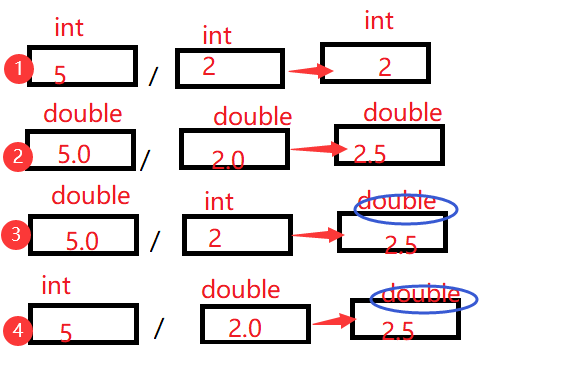`int i=289; char='a' c=i;`;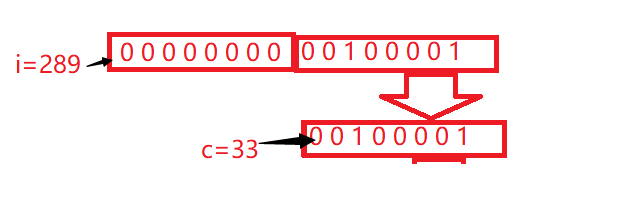## 四、输入和输出

### 4.1 标准文件

C 语言把所有的设备都当作文件。所以设备（比如显示器）被处理的方式与文件相同。以下三个文件会在程序执行时自动打开，以便访问键盘和屏幕。

C 语言中的 I/O (输入/输出) 通常使用 printf() 和 scanf() 两个函数。
scanf() 函数用于从标准输入（键盘）读取并格式化， printf() 函数发送格式化输出到标准输出（屏幕）。

1)所有的 C 语言程序都需要包含 main() 函数。 代码从 main() 函数开始执行。
2)printf() 用于格式化输出到屏幕。printf() 函数在 “stdio.h” 头文件中声明。
3)stdio.h 是一个头文件 (标准输入输出头文件) and #include 是一个预处理命令，用来引入头文件。 当编译器遇到 printf() 函数时，如果没有找到 stdio.h 头文件，会发生编译错误。
4)return 0; 语句用于表示退出程序。

### 4.2printf() 函数

#### 1)printf函数一般形式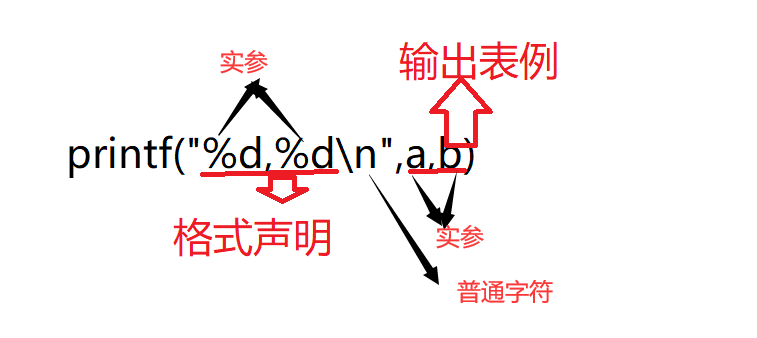#### 2)格式字符

12(12前面有3个空格)

##### 2)c格式符

-一个整数，如果在0~127范围中，也可以用“%%%%c”使之按字符形式输出，在输出前，系统会将该整数作为ASCII码转换成相应的字符;如`short a= 121;printf(" %%%%c ,a);`输出字符y

##### 4)f格式符

###### 1)基本型，用%%%%f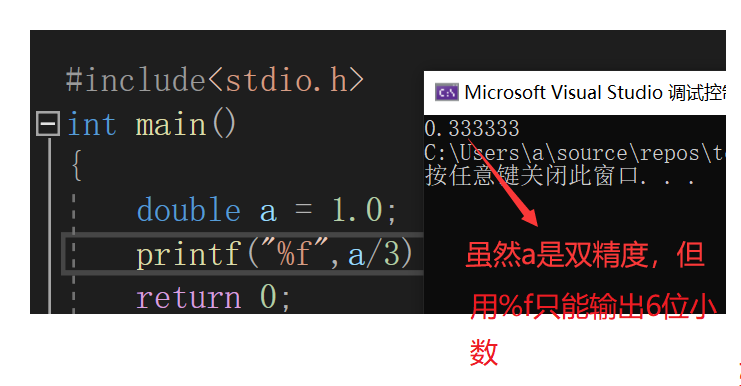###### 2)指定数据宽度和小数位数，用%%%%m.nf。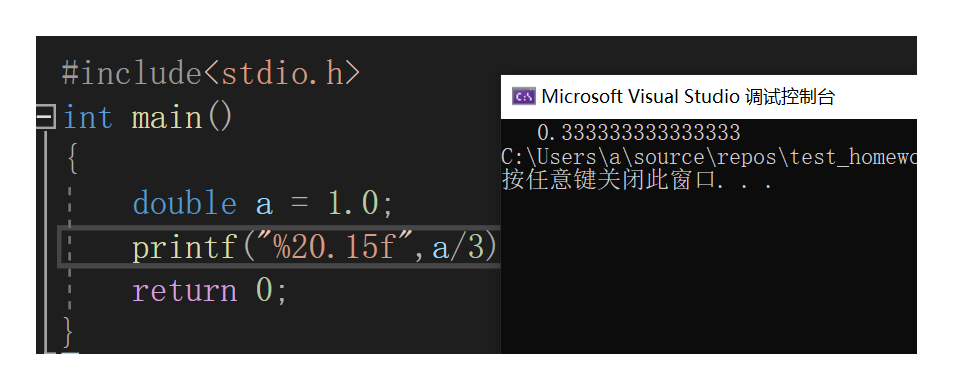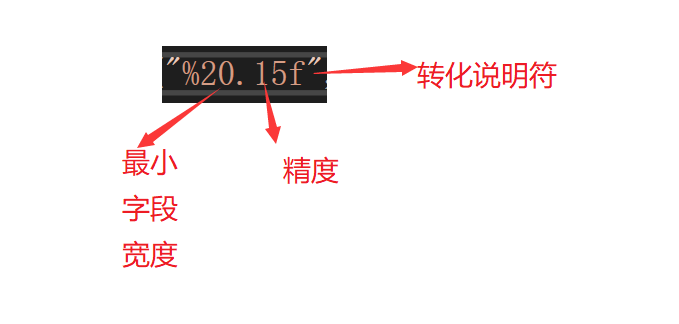###### 3）输出的数据向，左对齐用%%%%-m.nf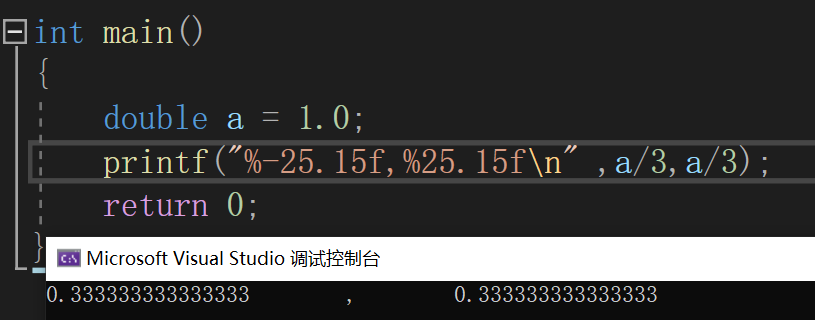###### 5)e格式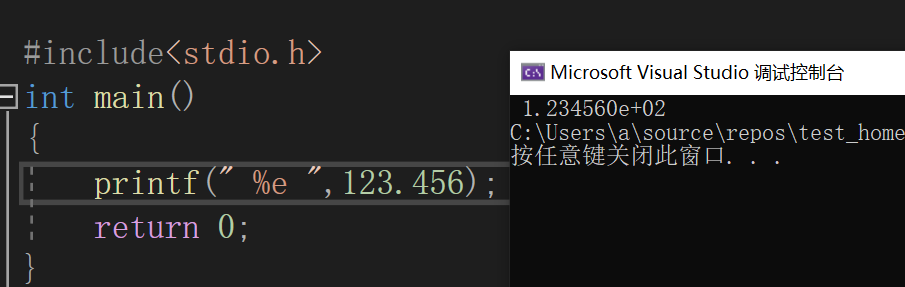##### 5)printf函数用的格式字符

%%%%d按整型数据的实际长度输出。
%%%%mdm为指定的输出字段的宽度。
%%%%ld输出长整型数据。
o以八进制整型式输出整数。
x以十六进制数形式输出整数。
u用来输出unsigned型数据，即无符号数，以十进制形式输出。
c用来输出一个字符。
s用来输出一个字符串。
f用来输出实数（包括单双精度），以小数形式输出。
e以指数形式输出实数。
g用来输出实数，它根据数值的大小，自动选f格式或e格式（选择输出是占宽度较小的一种），且不输出无意义的零。
p用于变量地址的输出。

### 4.3scanf函数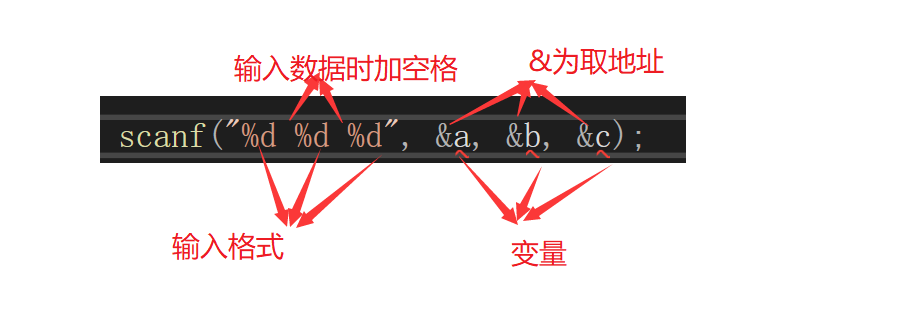1）scanf 函数中的格式控制后面应当是变量地址，而不是变量名
2）如果在格式控制字符串中除了格式声明以外还有其他字符,则在输人数据时在对应的位置上应输人与这些字符相同的字符。
3）在用“%%%%c”格式声明输人字符时，空格字符和“转义字符”中的字符都作为有效字符输入
4）)在输人数值数据时，如输人空格、回车.Tab键或遇非法宇字符(不属于数值的字符）认为该数据结束。

### 4.4getchar() & putchar() 函数

int getchar() 函数从屏幕读取下一个可用的字符，并把它返回为一个整数。这个函数在同一个时间内只会读取一个单一的字符。您可以在循环内使用这个方法，以便从屏幕上读取多个字符。

int putchar(int c) 函数把字符输出到屏幕上，并返回相同的字符。这个函数在同一个时间内只会输出一个单一的字符。您可以在循环内使用这个方法，以便在屏幕上输出多个字符。

## 五、END